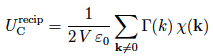# Question about ewald.cpp in LAMMPS

I don’t think the 1/2 is ever included due to the fact that only half the lattice vectors are used in LAMMPS–it is not needed. This is a trick that speeds up the calculation and is similar to using a half vs full neighbor list. The sum in the equation you referenced assumes that all the lattice vectors are used, so it is necessary to divide by 1/2.

Stan